# A simple CNN for the MNIST datasets – II – building the CNN with Keras and a first test

I continue with my series on first exploratory steps with CNNs. After all the discussion of CNN basics in the last article,

A simple CNN for the MNIST datasets – I,

we are well prepared to build a very simple CNN with Keras. By simple I mean simple enough to handle the MNIST digit images. The Keras API for creating CNN models, layers and activation functions is very convenient; a simple CNN does not require much code. So, the Jupyter environment is sufficient for our first experiment.

An interesting topic is the use of a GPU. After a somewhat frustrating experience with a MLP on the GPU of a NV 960 GTX in comparison to a i7 6700K CPU I am eager to see whether we get a reasonable GPU acceleration for a CNN. So, we should prepare our code to use the GPU. This requires a bit of preparation.

We should also ask a subtle question: What do we expect from a CNN in comparison to a MLP regarding the MNIST data? A MLP with 2 hidden layers (with 70 and 30 nodes) provided over 99.5% accuracy on the training data and almost 98% accuracy on a test dataset after some tweaking. Even with basic settings for our MLP we arrived at a value over 97.7% after 35 epochs - below 8 secs. Well, a CNN is probably better in feature recognition than a cluster detection algorithm. But we are talking about the last 2 % of remaining accuracy. I admit that I did not know what to expect ...

# A MLP as an important part of a CNN

At the end of the last article I had discussed a very simple layer structure of convolutional and pooling layers:

• Layer 0: Input layer (tensor of original image data, 3 layers for color channels or one layer for a gray channel)
• Layer 1: Conv layer (small 3x3 kernel, stride 1, 32 filters => 32 maps (26x26), overlapping filter areas)
• Layer 2: Pooling layer (2x2 max pooling => 32 (13x13) maps,
a map node covers 4x4 non overlapping areas per node on the original image)
• Layer 3: Conv layer (3x3 kernel, stride 1, 64 filters => 64 maps (11x11),
a map node covers 8x8 overlapping areas on the original image (total effective stride 2))
• Layer 4: Pooling layer (2x2 max pooling => 64 maps (5x5),
a map node covers 10x10 areas per node on the original image (total effective stride 5), some border info lost)
• Layer 5: Conv layer (3x3 kernel, stride 1, 64 filters => 64 maps (3x3),
a map node covers 18x18 areas per node (effective stride 5), some border info lost )

This is the CNN structure we are going to use in the near future. (Actually, I followed a suggestion of Francois Chollet; see the literature list in the last article). Let us assume that we somehow have established all these convolution and pooling layers for a CNN. Each layer producse some "feature"-related output, structured in form of a tensors. This led to an open question at the end of the last article:

Where and by what do we get a classification of the resulting data with respect to the 10 digit categories of the MNIST images?

Applying filters and extracting "feature hierarchies" of an image alone does not help without a "learned" judgement about these data. But the answer is very simple:

Use a MLP after the last Conv layer and feed it with data from this Conv layer!

When we think in terms of nodes and artificial neurons, we could say: We just have to connect the "nodes" of the feature maps of layer 5 in our special CNN with the nodes of an input layer of a MLP. As a MLP has a flat input layer we need to prepare 9x64 = 576 receiving "nodes" there. We would use weights with a value of "1.0" along these special connections.

Mathematically, this approach can be expressed in terms of a "flattening" operation on the tensor data produced by the the last Conv data. In Numpy terms: We need to reshape the multidimensional tensor containing the values across the stack of maps at the last Conv2D layer into a long 1D array (= a vector).

From a more general perspective we could say: Feeding the output of the Conv part of our CNN into a MLP for classification is quite similar to what we did when we pre-processed the MNIST data by an unsupervised cluster detection algorithm; also there we used the resulting data as input to an MLP. There is one big difference, however:

The optimization of the network's weights during training requires a BW propagation of error terms (more precisely: derivatives of the CNN's loss function) across the MLP AND the convolutional and pooling layers. Error BW propagation should not be stopped at the MLP's input layer: It has to move from the output layer of the MLP back to the MLP's input layer and from there to the convolutional and pooling layers. Remember that suitable filter kernels have to be found during (supervised) training.

If you read my PDF on the error back propagation for a MLP
PDF on the math behind Error Back_Propagation
and think a bit about its basic recipes and results you quickly see that the "input layer" of the MLP is no barrier to error back propagation: The "deltas" discussed in the PDF can be back-propagated right down to the MLP's input layer. Then we just apply the chain rule again. The partial derivatives at the nodes of the input layer with respect to their input values are just "1", because the activation function there is the identity function. The "weights" between the last Conv layer and the input layer of the MLP are no free parameters - we do not need to care about them. And then everything goes its normal way - we apply chain rule after chain rule for all nodes of the maps to determine the gradients of the CNN's loss function with respect to the weights there. But you need not think about the details - Keras and TF2 will take proper care about everything ...

But, you should always keep the following in mind: Whatever we discuss in terms of layers and nodes - in a CNN these are only fictitious interpretations of a series of mathematical operations on tensor data. Not less, not more ..,. Nodes and layers are just very helpful (!) illustrations of non-cyclic graphs of mathematical operations. KI on the level of my present discussion (MLPs, CNNs) "just" corresponds to algorithms which emerge out of a specific deterministic approach to solve an optimization problem.

# Using Tensorflow 2 and Keras

Let us now turn to coding. To be able to use a Nvidia GPU we need a Cuda/Cudnn installation and a working Tensorflow backend for Keras. I have already described the installation of CUDA 10.2 and CUDNN on an Opensuse Linux system in some detail in the article Getting a Keras based MLP to run with Cuda 10.2, Cudnn 7.6 and TensorFlow 2.0 on an Opensuse Leap 15.1 system. You can follow the hints there. In case you run into trouble on your Linux distribution try everything with Cuda 10.1.

Some more hints: TF2 in version 2.2 can be installed by the Pip-mechanism in your virtual Python environment ("pip install --upgrade tensorflow"). TF2 contains already a special Keras version - which is the one we are going to use in our upcoming experiment. So, there is no need to install Keras separately with "pip". Note also that, in contrast to TF1, it is NOT necessary to install a separate package "tensorflow-gpu". If all these things are new to you: You find some articles on creating an adequate ML test and development environment based on Python/PyDev/Jupyter somewhere else in this blog.

# Imports and settings for CPUs/GPUs

We shall use a Jupyter notebook to perform the basic experiments; but I recommend strongly to consolidate your code in Python files of an Eclipse/PyDev environment afterwards. Before you start your virtual Python environment from a Linux shell you should set the following environment variables:

```\$>export OPENBLAS_NUM_THREADS=4 # or whatever is reasonable for your CPU (but do not use all CPU cores and/or hyper threads
\$>export OMP_NUM_THREADS=4
\$>export TF_XLA_FLAGS=--tf_xla_cpu_global_jit
\$>export XLA_FLAGS=--xla_gpu_cuda_data_dir=/usr/local/cuda
\$>source bin/activate
(ml_1) \$> jupyter notebook
```

# Required Imports

The following commands in a first Jupyter cell perform the required library imports:

```import numpy as np
import scipy
import time
import sys
import os

import tensorflow as tf
from tensorflow import keras as K
from tensorflow.python.keras import backend as B
from keras import models
from keras import layers
from keras.utils import to_categorical
from keras.datasets import mnist
from tensorflow.python.client import device_lib

from sklearn.preprocessing import StandardScaler
```

Do not ignore the statement "from tensorflow.python.keras import backend as B"; we need it later.

The "StandardScaler" of Scikit-Learn will help us to "standardize" the MNIST input data. This is a step which you should know already from MLPs ... You can, of course, also experiment with different normalization procedures. But in my opinion using the "StandardScaler" is just convenient. ( I assume that you already have installed scikit-learn in your virtual Python environment).

# Settings for CPUs/GPUs

With TF2 the switching between CPU and GPU is a bit of a mess. Not all new parameters and their settings work as expected. As I have explained in the article on the Cuda installation named above, I, therefore, prefer to an old school, but reliable TF1 approach and use the compatibility interface:

```#gpu = False
gpu = True
if gpu:
GPU = True;  CPU = False; num_GPU = 1; num_CPU = 1
else:
GPU = False; CPU = True;  num_CPU = 1; num_GPU = 0

config = tf.compat.v1.ConfigProto(intra_op_parallelism_threads=6,
inter_op_parallelism_threads=1,
allow_soft_placement=True,
device_count = {'CPU' : num_CPU,
'GPU' : num_GPU},
log_device_placement=True

)
config.gpu_options.per_process_gpu_memory_fraction=0.35
config.gpu_options.force_gpu_compatible = True
B.set_session(tf.compat.v1.Session(config=config))

```

We are brave and try our first runs directly on a GPU. The statement "log_device_placement" will help us to get information about which device - CPU or GP - is actually used.

# Loading and preparing MNIST data

We prepare a function which loads and prepares the MNIST data for us. The statements reflect more or less what we did with the MNIST dat when we used them for MLPs.

```
# load MNIST
# **********
def load_Mnist():
mnist = K.datasets.mnist
(X_train, y_train), (X_test, y_test) = mnist.load_data()

#print(X_train.shape)
#print(X_test.shape)

# preprocess - flatten
len_train =  X_train.shape
len_test  =  X_test.shape
X_train = X_train.reshape(len_train, 28*28)
X_test  = X_test.reshape(len_test, 28*28)

#concatenate
_X = np.concatenate((X_train, X_test), axis=0)
_y = np.concatenate((y_train, y_test), axis=0)

_dim_X = _X.shape

# 32-bit
_X = _X.astype(np.float32)
_y = _y.astype(np.int32)

# normalize
scaler = StandardScaler()
_X = scaler.fit_transform(_X)

# mixing the training indices - MUST happen BEFORE encoding
shuffled_index = np.random.permutation(_dim_X)
_X, _y = _X[shuffled_index], _y[shuffled_index]

# split again
num_test  = 10000
num_train = _dim_X - num_test
X_train, X_test, y_train, y_test = _X[:num_train], _X[num_train:], _y[:num_train], _y[num_train:]

# reshape to Keras tensor requirements
train_imgs = X_train.reshape((num_train, 28, 28, 1))
test_imgs  = X_test.reshape((num_test, 28, 28, 1))
#print(train_imgs.shape)
#print(test_imgs.shape)

# one-hot-encoding
train_labels = to_categorical(y_train)
test_labels  = to_categorical(y_test)
#print(test_labels)

return train_imgs, test_imgs, train_labels, test_labels

if gpu:
with tf.device("/GPU:0"):
train_imgs, test_imgs, train_labels, test_labels= load_Mnist()
else:
with tf.device("/CPU:0"):
train_imgs, test_imgs, train_labels, test_labels= load_Mnist()

```

Some comments:

• Normalization and shuffling: The "StandardScaler" is used for data normalization. I also shuffled the data to avoid any pre-ordered sequences. We know these steps already from the MLP code we built in another article series.
• Image data in tensor form: Something, which is different from working with MLPs is that we have to fulfill some requirements regarding the form of input data. From the last article we know already that our data should have a tensor compatible form; Keras expects data from us which have a certain shape. So, no flattening of the data into a vector here as we were used to with MLPs. For images we, instead, need the width, the height of our images in terms of pixels and also the depth (here 1 for gray-scale images). In addition the data samples are to be indexed along the first tensor axis.
This means that we need to deliver a 4-dimensional array corresponding to a TF tensor of rank 4. Keras/TF2 will do the necessary transformation from a Numpy array to a TF2 tensor automatically for us. The corresponding Numpy shape of the required array is:
(samples, height, width, depth)
Some people also use the term "channels" instead of "depth". In the case of MNIST we reshape the input array - "train_imgs" to (num_train, 28, 28, 1), with "num_train" being the number of samples.
• The use of the function "to_categorical()", more precisely "tf.keras.utils.to_categorical()", corresponds to a one-hot-encoding of the target data. All these concepts are well known from our study of MLPs and MNIST. Keras makes life easy regarding this point ...
• The statements "with tf.device("/GPU:0"):" and "with tf.device("/CPU:0"):" delegate the execution of (suitable) code on the GPU or the CPU. Note that due to the Python/Jupyter environment some code will of course also be executed on the CPU - even if you delegated execution to the GPU.

If you activate the print statements the resulting output should be:

```(60000, 28, 28)
(10000, 28, 28)
(60000, 28, 28, 1)
(10000, 28, 28, 1)
[0. 0. 0. 0. 0. 0. 0. 1. 0. 0.]
```

The last line proves the one-hot-encoding.

# The CNN architecture - and Keras' layer API

Now, we come to a central point: We need to build the 5 central layers of our CNN-architecture. When we build our own MLP code we used a special method to build the different weight arrays, which represented the number of nodes via the array dimensions. A simple method was sufficient as we had no major differences between layers. But with CNNs we have to work with substantially different types of layers. So, how are layers to be handled with Keras?

Well, Keras provides a full layer API with different classes for a variety of layers. You find substantial information on this API and different types of layers at
https://keras.io/api/layers/.

The first section which is interesting for our experiment is https://keras.io/api/ layers/ convolution_layers/ convolution2d/.
You do not need to read much to understand that this is exactly what we need for the "convolutional layers" of our simple CNN model. But how do we instantiate the Conv2D class such that the output works seamlessly together with other layers?

Keras makes our life easy again. All layers are to be used in a purely sequential order. (There are much more complicated layer topologies you can build with Keras! Oh, yes ...). Well, you guess it: Keras offers you a model API; see:
https://keras.io/api/models/.

And there we find a class for a "sequential model" - see https://keras.io/api/ models/sequential/. This class offers us a method "add()" to add layers (and create an instance of the related layer class).

The only missing ingredient is a class for a "pooling" layer. Well, you find it in the layer API documentation, too. The following image depicts the basic structure of our CNN (see the left side of the drawing), as we designed it (see the list above):

# Keras code for the Conv and pooling layers

The convolutional part of the CNN can be set up by the following commands:

Convolutional part of the CNN

```# Sequential layer model of our CNN
# ***********************************

# Build the Conv part of the CNN
# ~~~~~~~~~~~~~~~~~~~~~~~~~~~~~~~

# Choose the activation function for the Conv2D layers
conv_act_func = 1
li_conv_act_funcs = ['sigmoid', 'relu', 'elu', 'tanh']
cact = li_conv_act_funcs[conv_act_func]

# Build the Conv2D layers
cnn = models.Sequential()
cnn.add(layers.Conv2D(32, (3,3), activation=cact, input_shape=(28,28,1)))
cnn.add(layers.MaxPooling2D((2,2)))
cnn.add(layers.Conv2D(64, (3,3), activation=cact))
cnn.add(layers.MaxPooling2D((2,2)))
cnn.add(layers.Conv2D(64, (3,3), activation=cact))

```

Easy, isn't it? The nice thing about Keras is that it cares about the required tensor ranks and shapes itself; in a sequential model it evaluates the output of a already defined layer to guess the shape of the tensor data entering the next layer. Thus we have to define an "input_shape" only for data entering the first Conv2D layer!

The first Conv2D layer requires, of course, a shape for the input data. We must also tell the layer interface how many filters and "feature maps" we want to use. In our case we produce 32 maps by first Conv2D layer and 64 by the other two Conv2D layers. The (3x3)-parameter defines the filter area size to be covered by the filter kernel: 3x3 pixels. We define no "stride", so a stride of 1 is automatically used; all 3x3 areas lie close to each other and overlap each other. These parameters result in 32 maps of size 26x26 after the first convolution. The size of the maps of the other layers are given in the layer list at the beginning of this article.

In addition you saw from the code that we chose an activation function via an index of a Python list of reasonable alternatives. You find an explanation of all the different activation functions in the literature. (See also: wikipedia: Activation function). The "sigmoid" function should be well known to you already from my other article series on a MLP.

Now, we have to care about the MLP part of the CNN. The code is simple:

MLP part of the CNN

```# Build the MLP part of the CNN
# ~~~~~~~~~~~~~~~~~~~~~~~~~~~~~~~

# Choose the activation function for the hidden layers of the MLP
mlp_h_act_func = 0
li_mlp_h_act_funcs = ['relu', 'sigmoid', 'tanh']
mhact = li_mlp_h_act_funcs[mlp_h_act_func]

# Choose the output function for the output layer of the MLP
mlp_o_act_func = 0
li_mlp_o_act_funcs = ['softmax', 'sigmoid']
moact = li_mlp_o_act_funcs[mlp_o_act_func]

# Build the MLP layers
cnn.add(layers.Flatten())
cnn.add(layers.Dense(70, activation=mhact))
#cnn.add(layers.Dense(30, activation=mhact))
cnn.add(layers.Dense(10, activation=moact))

```

This all is very straight forward (with the exception of the last statement). The "Flatten"-layer corresponds to the MLP's inout layer. It just transforms the tensor output of the last Conv2D layer into the flat form usable for the first "Dense" layer of the MLP. The first and only "Dense layer" (MLP hidden layer) builds up connections from the flat MLP "input layer" and associates it with weights. Actually, it prepares a weight-tensor for a tensor-operation on the output data of the feeding layer. Dense means that all "nodes" of the previous layer are connected to the present layer's own "nodes" - meaning: setting the right dimensions of the weight tensor (matrix in our case). As a first trial we work with just one hidden layer. (We shall later see that more layers will not improve accuracy.)

I choose the output function (if you will: the activation function of the output layer) as "softmax". This gives us a probability distribution across the classification categories. Note that this is a different approach compared to what we have done so far with MLPs. I shall comment on the differences in a separate article when I find the time for it. At this point I just want to indicate that softmax combined with the "categorical cross entropy loss" is a generalized version of the combination "sigmoid" with "log loss" as we used it for our MLP.

# Parameterization

The above code for creating the CNN would work. However, we want to be able to parameterize our simple CNN. So we include the above statements in a function for which we provide parameters for all layers. A quick solution is to define layer parameters as elements of a Python list - we then get one list per layer. (If you are a friend of clean code design I recommend to choose a more elaborated approach; inject just one parameter object containing all parameters in a structured way. I leave this exercise to you.)

We now combine the statements for layer construction in a function:

```
# Sequential layer model of our CNN
# ***********************************

# just for illustration - th ereal parameters are fed later
# ~~~~~~~~~~~~~~~~~~~~~~~~~~~~~~~~~~~~~~~~~~~~~~~~~~~~~~~~~
li_conv_1   = [32, (3,3), 0]
li_conv_2   = [64, (3,3), 0]
li_conv_3   = [64, (3,3), 0]
li_Conv     = [li_conv_1, li_conv_2, li_conv_3]
li_pool_1   = [(2,2)]
li_pool_2   = [(2,2)]
li_Pool     = [li_pool_1, li_pool_2]
li_dense_1  = [70, 0]
li_dense_2  = [10, 0]
li_MLP      = [li_dense_1, li_dense_2]
input_shape = (28,28,1)

# important !!
# ~~~~~~~~~~~
cnn = None

def build_cnn_simple(li_Conv, li_Pool, li_MLP, input_shape ):

# activation functions to be used in Conv-layers
li_conv_act_funcs = ['relu', 'sigmoid', 'elu', 'tanh']
# activation functions to be used in MLP hidden layers
li_mlp_h_act_funcs = ['relu', 'sigmoid', 'tanh']
# activation functions to be used in MLP output layers
li_mlp_o_act_funcs = ['softmax', 'sigmoid']

# Build the Conv part of the CNN
# ~~~~~~~~~~~~~~~~~~~~~~~~~~~~~~~
num_conv_layers = len(li_Conv)
num_pool_layers = len(li_Pool)
if num_pool_layers != num_conv_layers - 1:
print("\nNumber of pool layers does not fit to number of Conv-layers")
sys.exit()
rg_il = range(num_conv_layers)

# Define a sequential model
cnn = models.Sequential()

for il in rg_il:
# add the convolutional layer
num_filters = li_Conv[il]
t_fkern_size = li_Conv[il]
cact        = li_conv_act_funcs[li_Conv[il]]
if il==0:
cnn.add(layers.Conv2D(num_filters, t_fkern_size, activation=cact, input_shape=input_shape))
else:
cnn.add(layers.Conv2D(num_filters, t_fkern_size, activation=cact))

# add the pooling layer
if il < num_pool_layers:
t_pkern_size = li_Pool[il]
cnn.add(layers.MaxPooling2D(t_pkern_size))

# Build the MLP part of the CNN
# ~~~~~~~~~~~~~~~~~~~~~~~~~~~~~~~
num_mlp_layers = len(li_MLP)
rg_im = range(num_mlp_layers)

cnn.add(layers.Flatten())

for im in rg_im:
# add the dense layer
n_nodes = li_MLP[im]
if im < num_mlp_layers - 1:
m_act   =  li_mlp_h_act_funcs[li_MLP[im]]
else:
m_act   =  li_mlp_o_act_funcs[li_MLP[im]]
cnn.add(layers.Dense(n_nodes, activation=m_act))

return cnn

```

We return the model "cnn" to be able to use it afterwards.

# How many parameters does our CNN have?

The layers contribute with the following numbers of weight parameters:

• Layer 1: (32 x (3x3)) + 32 = 320
• Layer 3: 32 x 64 x (3x3) + 64 = 18496
• Layer 5: 64 x 64 x (3x3) + 64 = 36928
• MLP : (576 + 1) x 70 + (70 + 1) x 10 = 41100

Making a total of 96844 weight parameters. Our standard MLP discussed in another article series had (784+1) x 70 + (70 + 1) x 30 + (30 +1 ) x 10 = 57390 weights. So, our CNN is bigger and the CPU time to follow and calculate all the partial derivatives will be significantly higher. So, we should definitely expect some better classification data, shouldn't we?

# Compilation

Now comes a thing which is necessary for models: We have not yet defined the loss function and the optimizer or a learning rate. For the latter Keras can choose a proper value itself - as soon as it knows the loss function. But we should give it a reasonable loss function and a suitable optimizer for gradient descent. This is the main purpose of the "compile()"-function.

```cnn.compile(optimizer='rmsprop', loss='categorical_crossentropy', metrics=['accuracy'])
```

Although TF2 can already analyze the graph of tensor operations for partial derivatives, it cannot guess the beginning of the chain rule sequence.

As we have multiple categories "categorial_crossentropy" is a good choice for the loss function. We should also define which optimized gradient descent method is used; we choose "rmsprop" - as this method works well in most cases. A nice introduction is given here: towardsdatascience: understanding-rmsprop-faster-neural-network-learning-62e116fcf29a. But see the books mentioned in the last article on "rmsprop", too.

Regarding the use of different metrics for different tasks see machinelearningmastery.com / custom-metrics-deep-learning-keras-python/. In case of a classification problem, we are interested in the categorical "accuracy". A metric can be monitored during training and will be recorded (besides aother data). We can use it for plotting information on the training process (a topic of the next article).

# Training

Training is done by a function model.fit() - here: cnn.fit(). This function accepts a variety of parameters explained here: https://keras.io/ api/ models/ model_training_apis/ #fit-method.

We now can combine compilation and training in one function:

```# Training
def train( cnn, build=False, train_imgs, train_labels, reset, epochs, batch_size, optimizer, loss, metrics,
li_Conv, li_Poo, li_MLP, input_shape ):
if build:
cnn = build_cnn_simple( li_Conv, li_Pool, li_MLP, input_shape)
cnn.compile(optimizer=optimizer, loss=loss, metrics=metrics)
cnn.save_weights('cnn_weights.h5') # save the initial weights
# reset weights
if reset and not build:
cnn.load_weights('cnn_weights.h5')
start_t = time.perf_counter()
cnn.fit(train_imgs, train_labels, epochs=epochs, batch_size=batch_size, verbose=1, shuffle=True)
end_t = time.perf_counter()
fit_t = end_t - start_t
return cnn, fit_t  # we return cnn to be able to use it by other functions
```

Note that we save the initial weights to be able to load them again for a new training - otherwise Keras saves the weights as other model data after training and continues with the last weights found. The latter can be reasonable if you want to continue training in defined steps. However, in our forthcoming tests we repeat the training from scratch.

Keras offers a "save"-model and methods to transfer data of a CNN model to files (in two specific formats). For saving weights the given lines are sufficient. Hint: When I specify no path to the file "cnn_weights.h5" the data are - at least in my virtual Python environment - saved in the directory where the notebook is located.

# First test

In a further Jupyter cell we place the following code for a test run:

```
# Perform a training run
# ********************
build = False
if cnn == None:
build = True
batch_size=64
epochs=5
reset = True # we want training to start again with the initial weights

optimizer='rmsprop'
loss='categorical_crossentropy'
metrics=['accuracy']

li_conv_1   = [32, (3,3), 0]
li_conv_2   = [64, (3,3), 0]
li_conv_3   = [64, (3,3), 0]
li_Conv     = [li_conv_1, li_conv_2, li_conv_3]
li_pool_1   = [(2,2)]
li_pool_2   = [(2,2)]
li_Pool     = [li_pool_1, li_pool_2]
li_dense_1  = [70, 0]
li_dense_2  = [10, 0]
li_MLP      = [li_dense_1, li_dense_2]
input_shape = (28,28,1)

try:
if gpu:
with tf.device("/GPU:0"):
cnn, fit_time = train( cnn, build, train_imgs, train_labels,
reset, epochs, batch_size, optimizer, loss, metrics,
li_Conv, li_Pool, li_MLP, input_shape)
print('Time_GPU: ', fit_time)
else:
with tf.device("/CPU:0"):
cnn, fit_time = train( cnn, build, train_imgs, train_labels,
reset, epochs, batch_size, optimizer, loss, metrics,
li_Conv, li_Pool, li_MLP, input_shape)
print('Time_CPU: ', fit_time)
except SystemExit:
print("stopped due to exception")
```

You recognize the parameterization of our train()-function. What results do we get ?

```Epoch 1/5
60000/60000 [==============================] - 4s 69us/step - loss: 0.1551 - accuracy: 0.9520
Epoch 2/5
60000/60000 [==============================] - 4s 69us/step - loss: 0.0438 - accuracy: 0.9868
Epoch 3/5
60000/60000 [==============================] - 4s 68us/step - loss: 0.0305 - accuracy: 0.9907
Epoch 4/5
60000/60000 [==============================] - 4s 69us/step - loss: 0.0227 - accuracy: 0.9931
Epoch 5/5
60000/60000 [==============================] - 4s 69us/step - loss: 0.0184 - accuracy: 0.9948
Time_GPU:  20.610678611003095
```

And a successive check on the test data gives us: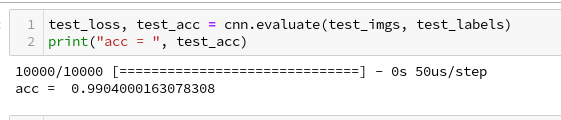We can ask Keras also for a description of the model:

# Accuracy at the 99% level

We got an accuracy on the test data of 99%! With 5 epochs in 20 seconds - on my old GPU.
This leaves us a very good impression - on first sight ...

# Conclusion

We saw today that it is easy to set up a CNN. We used a simple MLP to solve the problem of classification; the data to its input layer are provided by the output of the last convolutional layer. The tensor there has just to be "flattened".

The level of accuracy reached is impressing. Well, its also a bit frustrating when we think about the efforts we put into our MLP, but we also get a sense for the power and capabilities of CNNs.

In the next article
A simple CNN for the MNIST dataset – III – inclusion of a learning-rate scheduler, momentum and a L2-regularizer
we will care a bit about plotting. We at least want to see the same accuracy and loss data which we used to plot at the end of our MLP tests.

# MLP, Numpy, TF2 – performance issues – Step III – a correction to BW propagation

In the last articles of this series

we looked at the FW-propagation of the MLP code which I discussed in another article series. We found that the treatment of bias neurons in the input layer was technically inefficient due to a collision of C- and F-contiguous arrays. By circumventing the problem we could accelerate the FW-propagation of big batches (as the complete training or test data set) by more than a factor of 2.

In this article I want to turn to the BW propagation and do some analysis regarding CPU consumption there. We will find a simple (and stupid) calculation step there which we shall replace. This will give us another 15% to 22% performance improvement in comparison to what we have reached in the last article for MNIST data:

• 9.6 secs for 35 epochs and a batch-size of 500
• and 8.7 secs for a batch-size of 20000.

# Present CPU time relation between the FW- and the BW-propagation

The central training of mini-batches is performed by the method "_handle_mini_batch()".

```#
''' -- Method to deal with a batch -- '''
def _handle_mini_batch (self, num_batch = 0, num_epoch = 0, b_print_y_vals = False, b_print = False, b_keep_bw_matrices = True):
''' .... '''
# Layer-related lists to be filled with 2-dim Numpy matrices during FW propagation
# ********************************************************************************
li_Z_in_layer  = [None] * self._n_total_layers # List of matrices with z-input values for each layer; filled during FW-propagation
li_A_out_layer = li_Z_in_layer.copy()          # List of matrices with results of activation/output-functions for each layer; filled during FW-propagation
li_delta_out   = li_Z_in_layer.copy()          # Matrix with out_delta-values at the outermost layer
li_delta_layer = li_Z_in_layer.copy()          # List of the matrices for the BW propagated delta values
li_D_layer     = li_Z_in_layer.copy()          # List of the derivative matrices D containing partial derivatives of the activation/ouput functions
li_grad_layer  = li_Z_in_layer.copy()          # List of the matrices with gradient values for weight corrections

# Major steps for the mini-batch during one epoch iteration
# **********************************************************

#ts=time.perf_counter()
# Step 0: List of indices for data records in the present mini-batch
# ******
ay_idx_batch = self._ay_mini_batches[num_batch]

# Step 1: Special preparation of the Z-input to the MLP's input Layer L0
# ******
# ts=time.perf_counter()
# slicing
li_Z_in_layer = self._X_train[ay_idx_batch] # numpy arrays can be indexed by an array of integers

# transposition
#~~~~~~~~~~~~~~
li_Z_in_layer = li_Z_in_layer.T
#te=time.perf_counter(); t_batch = te - ts;
#print("\nti - transposed inputbatch =", t_batch)

# Step 2: Call forward propagation method for the present mini-batch of training records
# *******
#tsa = time.perf_counter()
self._fw_propagation(li_Z_in = li_Z_in_layer, li_A_out = li_A_out_layer)
#tea = time.perf_counter(); ta = tea - tsa;  print("ta - FW-propagation", "%10.8f"%ta)

# Step 3: Cost calculation for the mini-batch
# ********
#tsb = time.perf_counter()
ay_y_enc = self._ay_onehot[:, ay_idx_batch]
ay_ANN_out = li_A_out_layer[self._n_total_layers-1]
total_costs_batch, rel_reg_contrib = self._calculate_loss_for_batch(ay_y_enc, ay_ANN_out, b_print = False)
# we add the present cost value to the numpy array
self._ay_costs[num_epoch, num_batch]            = total_costs_batch
self._ay_reg_cost_contrib[num_epoch, num_batch] = rel_reg_contrib
#teb = time.perf_counter(); tb = teb - tsb; print("tb - cost calculation", "%10.8f"%tb)

# Step 4: Avg-error for later plotting
# ********
#tsc = time.perf_counter()
# mean "error" values - averaged over all nodes at outermost layer and all data sets of a mini-batch
ay_theta_out = ay_y_enc - ay_ANN_out
ay_theta_avg = np.average(np.abs(ay_theta_out))
self._ay_theta[num_epoch, num_batch] = ay_theta_avg
#tec = time.perf_counter(); tc = tec - tsc; print("tc - error", "%10.8f"%tc)

# Step 5: Perform gradient calculation via back propagation of errors
# *******
#tsd = time.perf_counter()
self._bw_propagation( ay_y_enc = ay_y_enc,
li_Z_in = li_Z_in_layer,
li_A_out = li_A_out_layer,
li_delta_out = li_delta_out,
li_delta = li_delta_layer,
li_D = li_D_layer,
li_grad = li_grad_layer,
b_print = b_print,
b_internal_timing = False
)
#ted = time.perf_counter(); td = ted - tsd; print("td - BW propagation", "%10.8f"%td)

# Step 7: Adjustment of weights
# *******
#tsf = time.perf_counter()
rg_layer=range(0, self._n_total_layers -1)
for N in rg_layer:
delta_w_N = self._learn_rate * li_grad_layer[N]
self._li_w[N] -= ( delta_w_N + (self._mom_rate * self._li_mom[N]) )

# save momentum
self._li_mom[N] = delta_w_N
#tef = time.perf_counter(); tf = tef - tsf; print("tf - weight correction", "%10.8f"%tf)

return None

```

I took some time measurements there:

```ti - transposed inputbatch = 0.0001785
ta - FW-propagation 0.00080975
tb - cost calculation 0.00030705
tc - error 0.00016182
td - BW propagation 0.00112558
tf - weight correction 0.00020079

ti - transposed inputbatch = 0.00018144
ta - FW-propagation 0.00082022
tb - cost calculation 0.00031284
tc - error 0.00016652
td - BW propagation 0.00106464
tf - weight correction 0.00019576
```

You see that the FW-propagation is a bit faster than the BW-propagation. This is a bit strange as the FW-propagation is dominated meanwhile by a really expensive operation which we cannot accelerate (without choosing a new activation function): The calculation of the sigmoid value for the inputs at layer L1.

So let us look into the BW-propagation; the code for it is momentarily:

```    ''' -- Method to handle error BW propagation for a mini-batch --'''
def _bw_propagation(self,
ay_y_enc, li_Z_in, li_A_out,
li_delta_out, li_delta, li_D, li_grad,
b_print = True, b_internal_timing = False):

# List initialization: All parameter lists or arrays are filled or to be filled by layer operations
# Note: the lists li_Z_in, li_A_out were already filled by _fw_propagation() for the present batch

# Initiate BW propagation - provide delta-matrices for outermost layer
# ***********************
tsa = time.perf_counter()
# Input Z at outermost layer E  (4 layers -> layer 3)
ay_Z_E = li_Z_in[self._n_total_layers-1]
# Output A at outermost layer E (was calculated by output function)
ay_A_E = li_A_out[self._n_total_layers-1]

# Calculate D-matrix (derivative of output function) at outmost the layer - presently only D_sigmoid
ay_D_E = self._calculate_D_E(ay_Z_E=ay_Z_E, b_print=b_print )
#ay_D_E = ay_A_E * (1.0 - ay_A_E)

# Get the 2 delta matrices for the outermost layer (only layer E has 2 delta-matrices)
ay_delta_E, ay_delta_out_E = self._calculate_delta_E(ay_y_enc=ay_y_enc, ay_A_E=ay_A_E, ay_D_E=ay_D_E, b_print=b_print)

# add the matrices to their lists ; li_delta_out gets only one element
idxE = self._n_total_layers - 1
li_delta_out[idxE] = ay_delta_out_E # this happens only once
li_delta[idxE]     = ay_delta_E
li_D[idxE]         = ay_D_E
li_grad[idxE]      = None    # On the outermost layer there is no gradient !

tea = time.perf_counter(); ta = tea - tsa; print("\nta-bp", "%10.8f"%ta)

# Loop over all layers in reverse direction
# ******************************************
# index range of target layers N in BW direction (starting with E-1 => 4 layers -> layer 2))
range_N_bw_layer = reversed(range(0, self._n_total_layers-1))   # must be -1 as the last element is not taken

# loop over layers
tsb = time.perf_counter()
for N in range_N_bw_layer:

# Back Propagation operations between layers N+1 and N
# *******************************************************
# this method handles the special treatment of bias nodes in Z_in, too
tsib = time.perf_counter()
ay_delta_N, ay_D_N, ay_grad_N = self._bw_prop_Np1_to_N( N=N, li_Z_in=li_Z_in, li_A_out=li_A_out, li_delta=li_delta, b_print=False )
teib = time.perf_counter(); tib = teib - tsib; print("N = ", N, " tib-bp", "%10.8f"%tib)

# add matrices to their lists
#tsic = time.perf_counter()
li_delta[N] = ay_delta_N
li_D[N]     = ay_D_N
li_grad[N]= ay_grad_N
#teic = time.perf_counter(); tic = teic - tsic; print("\nN = ", N, " tic = ", "%10.8f"%tic)
teb = time.perf_counter(); tb = teb - tsb; print("tb-bp", "%10.8f"%tb)

return
```

Typical timing results are:

```ta-bp 0.00007112
N =  2  tib-bp 0.00025399
N =  1  tib-bp 0.00051683
N =  0  tib-bp 0.00035941
tb-bp 0.00126436

ta-bp 0.00007492
N =  2  tib-bp 0.00027644
N =  1  tib-bp 0.00090043
N =  0  tib-bp 0.00036728
tb-bp 0.00168378
```

We see that the CPU consumption of "_bw_prop_Np1_to_N()" should be analyzed in detail. It is relatively time consuming at every layer, but especially at layer L1. (The list adds are insignificant.)
What does this method presently look like?

```    ''' -- Method to calculate the BW-propagated delta-matrix and the gradient matrix to/for layer N '''
def _bw_prop_Np1_to_N(self, N, li_Z_in, li_A_out, li_delta, b_print=False):
'''
BW-error-propagation between layer N+1 and N
Version 1.5 - partially accelerated

Inputs:
li_Z_in:  List of input Z-matrices on all layers - values were calculated during FW-propagation
li_A_out: List of output A-matrices - values were calculated during FW-propagation
li_delta: List of delta-matrices - values for outermost ölayer E to layer N+1 should exist

Returns:
ay_delta_N - delta-matrix of layer N (required in subsequent steps)
ay_D_N     - derivative matrix for the activation function on layer N
ay_grad_N  - matrix with gradient elements of the cost fnction with respect to the weights on layer N
'''

# Prepare required quantities - and add bias neuron to ay_Z_in
# ****************************

# Weight matrix meddling between layers N and N+1
ay_W_N = self._li_w[N]

# delta-matrix of layer N+1
ay_delta_Np1 = li_delta[N+1]

# fetch output value saved during FW propagation
ay_A_N = li_A_out[N]

# Optimization V1.5 !
if N > 0:

#ts=time.perf_counter()
ay_Z_N = li_Z_in[N]
# !!! Add intermediate row (for bias) to Z_N !!!
ay_Z_N = self._add_bias_neuron_to_layer(ay_Z_N, 'row')
#te=time.perf_counter(); t1 = te - ts; print("\nBW t1 = ", t1, " N = ", N)

# Derivative matrix for the activation function (with extra bias node row)
# ********************
#    can only be calculated now as we need the z-values
#ts=time.perf_counter()
ay_D_N = self._calculate_D_N(ay_Z_N)
#te=time.perf_counter(); t2 = te - ts; print("\nBW t2 = ", t2, " N = ", N)

# Propagate delta
# **************

# intermediate delta
# ~~~~~~~~~~~~~~~~~~
#ts=time.perf_counter()
ay_delta_w_N = ay_W_N.T.dot(ay_delta_Np1)
#te=time.perf_counter(); t3 = te - ts; print("\nBW t3 = ", t3)

# final delta
# ~~~~~~~~~~~
#ts=time.perf_counter()
ay_delta_N = ay_delta_w_N * ay_D_N

# Orig reduce dimension again
# ****************************
ay_delta_N = ay_delta_N[1:, :]
#te=time.perf_counter(); t4 = te - ts; print("\nBW t4 = ", t4)

else:
ay_delta_N = None
ay_D_N = None

# Calculate gradient
# ********************
#ts=time.perf_counter()
ay_grad_N = np.dot(ay_delta_Np1, ay_A_N.T)
#te=time.perf_counter(); t5 = te - ts; print("\nBW t5 = ", t5)

# regularize gradient (!!!! without adding bias nodes in the L1, L2 sums)
#ts=time.perf_counter()
if self._lambda2_reg > 0:
ay_grad_N[:, 1:] += self._li_w[N][:, 1:] * self._lambda2_reg
if self._lambda1_reg > 0:
ay_grad_N[:, 1:] += np.sign(self._li_w[N][:, 1:]) * self._lambda1_reg
#te=time.perf_counter(); t6 = te - ts; print("\nBW t6 = ", t6)

return ay_delta_N, ay_D_N, ay_grad_N

```

Timing data for a batch-size of 500 are:

```N =  2
BW t1 =  0.0001169009999557602  N =  2
BW t2 =  0.00035331499998392246  N =  2
BW t3 =  0.00018078099992635543
BW t4 =  0.00010234199999104021
BW t5 =  9.928200006470433e-05
BW t6 =  2.4267000071631628e-05
N =  2  tib-bp 0.00124414

N =  1
BW t1 =  0.0004323499999827618  N =  1
BW t2 =  0.000781415999881574  N =  1
BW t3 =  4.2077999978573644e-05
BW t4 =  0.00022921000004316738
BW t5 =  9.376399998473062e-05
BW t6 =  0.00012183700005152787
N =  1  tib-bp 0.00216281

N =  0
BW t5 =  0.0004289769999559212
BW t6 =  0.00015404999999191205
N =  0  tib-bp 0.00075249
....
N =  2
BW t1 =  0.00012802800006284087  N =  2
BW t2 =  0.00034988200013685855  N =  2
BW t3 =  0.0001854429999639251
BW t4 =  0.00010359299994888715
BW t5 =  0.00010210400000687514
BW t6 =  2.4010999823076418e-05
N =  2  tib-bp 0.00125854

N =  1
BW t1 =  0.0004407169999467442  N =  1
BW t2 =  0.0007845899999665562  N =  1
BW t3 =  0.00025684100000944454
BW t4 =  0.00012409999999363208
BW t5 =  0.00010345399982725212
BW t6 =  0.00012994100006835652
N =  1  tib-bp 0.00221321

N =  0
BW t5 =  0.00044504700008474174
BW t6 =  0.00016473000005134963
N =  0  tib-bp 0.00071442

....
N =  2
BW t1 =  0.000292730999944979  N =  2
BW t2 =  0.001102525000078458  N =  2
BW t3 =  2.9429999813146424e-05
BW t4 =  8.547999868824263e-06
BW t5 =  3.554099998837046e-05
BW t6 =  2.5041999833774753e-05
N =  2  tib-bp 0.00178565

N =  1
BW t1 =  3.143399999316898e-05  N =  1
BW t2 =  0.0006720640001276479  N =  1
BW t3 =  5.4785999964224175e-05
BW t4 =  9.756200006449944e-05
BW t5 =  0.0001605449999715347
BW t6 =  1.8391000139672542e-05
N =  1  tib-bp 0.00147566

N =  0
BW t5 =  0.0003641810001226986
BW t6 =  6.338999992294703e-05
N =  0  tib-bp 0.00046542

```

It seems that we should care about t1, t2, t3 for hidden layers and maybe about t5 at layers L1/L0.

However, for a batch-size of 15000 things look a bit different:

```N =  2
BW t1 =  0.0005776280000304723  N =  2
BW t2 =  0.004995969999981753  N =  2
BW t3 =  0.0003165199999557444
BW t4 =  0.0005244750000201748
BW t5 =  0.000518499999998312
BW t6 =  2.2458999978880456e-05
N =  2  tib-bp 0.00736144

N =  1
BW t1 =  0.0010120430000029046  N =  1
BW t2 =  0.010797029000002567  N =  1
BW t3 =  0.0005006920000028003
BW t4 =  0.0008704929999794331
BW t5 =  0.0010805200000163495
BW t6 =  3.0326000000968634e-05
N =  1  tib-bp 0.01463436

N =  0
BW t5 =  0.006987539000022025
BW t6 =  0.00023552499999368592
N =  0  tib-bp 0.00730959

N =  2
BW t1 =  0.0006299790000525718  N =  2
BW t2 =  0.005081416999985322  N =  2
BW t3 =  0.00018547400003399162
BW t4 =  0.0005970070000103078
BW t5 =  0.000564008000026206
BW t6 =  2.3311000006742688e-05
N =  2  tib-bp 0.00737899

N =  1
BW t1 =  0.0009376909999900818  N =  1
BW t2 =  0.010650266999959968  N =  1
BW t3 =  0.0005232729999988806
BW t4 =  0.0009100700000317374
BW t5 =  0.0011237720000281115
BW t6 =  0.00016643800000792908
N =  1  tib-bp 0.01466144

N =  0
BW t5 =  0.006987463000029948
BW t6 =  0.00023978600000873485
N =  0  tib-bp 0.00734308
```

For big batch-sizes "t2" dominates everything. It seems that we have found another code area which causes the trouble with big batch-sizes which we already observed before!

# What operations do the different CPU times stand for?

To keep an overview without looking into the code again, I briefly summarize which operations cause which of the measured time differences:

• "t1" - which contributes for small batch-sizes stands for adding a bias neuron to the input data Z_in at each layer.
• "t2" - which is by far dominant for big batch sizes stands for calculating the derivative of the output/activation function (in our case of the sigmoid function) at the various layers.
• "t3" - which contributes at some layers stands for a dot()-matrix multiplication with the transposed weight-matrix,
• "t4" - covers an element-wise matrix-multiplication,
• "t5" - contributes at the BW-transition from layer L1 to L0 and covers the matrix multiplication there (including the full output matrix with the bias neurons at L0)

# Use the output values calculated at each layer during FW-propagation!

Why does the calculation of the derivative of the sigmoid function take so much time? Answer: Because I coded it stupidly! Just look at it:

```    ''' -- Method to calculate the matrix with the derivative values of the output function at outermost layer '''
def _calculate_D_N(self, ay_Z_N, b_print= False):
'''
This method calculates and returns the D-matrix for the outermost layer
The D matrix contains derivatives of the output function with respect to local input "z_j" at outermost nodes.

Returns
------
ay_D_E:    Matrix with derivative values of the output function
with respect to local z_j valus at the nodes of the outermost layer E
Note: This is a 2-dim matrix over layer nodes and training samples of the mini-batch
'''
if self._my_out_func == 'sigmoid':
ay_D_E = self._D_sigmoid(ay_Z = ay_Z_N)

else:
print("The derivative for output function " + self._my_out_func + " is not known yet!" )
sys.exit()

return ay_D_E

''' -- method for the derivative of the sigmoid function-- '''
def _D_sigmoid(self, ay_Z):
'''
Derivative of sigmoid function with respect to Z-values
- works via expit element-wise on matrices
Input:  Z - Matrix with Input values for the activation function Phi() = sigmoid()
Output: D - Matrix with derivative values
'''
S_Z = self._sigmoid(ay_Z)
return S_Z * (1.0 - S_Z)
```

We first call an intermediate function which then directs us to the right function for a chosen activation function. Well meant: So far, we use only the sigmoid function, but it could e.g. also be the relu() or tanh()-function. So, we did what we did for the sake of generalization. But we did it badly because of two reasons:

• We did not keep up a function call pattern which we introduced in the FW-propagation.
• The calculation of the derivative is inefficient.

The first point is a minor one: During FW-propagation we called the right (!) activation function, i.e. the one we choose by input parameters to our ANN-object, by an indirect call. Why not do it the same way here? We would avoid an intermediate function call and keep up a pattern. Actually, we prepared the necessary definitions already in the __init__()-function.

The second point is relevant for performance: The derivative function produces the correct results for a given "ay_Z", but this is totally inefficient in our BW-situation. The code repeats a really expensive operation which we have already performed during FW-propagation: calling sigmoid(ay_Z) to get "A_out"-values per layer then. We even put the A_out-values [=sigmoid(ay_Z_in)] per layer and batch (!) with some foresight into a list in "li_A_out[]" at that point of the code (see the FW-propagation code discussed in the last article).

So, of course, we should use these "A_out"-values now in the BW-steps! No further comment .... you see what we need to do.

Hint: Actually, also other activation functions "act(Z)" like e.g. the "tanh()"-function have derivatives which depend on on "A=act(Z)", only. So, we should provide Z and A via an interface to the derivative function and let the respective functions take what it needs.
But, my insight into my own dumbness gets worse.

# Eliminate the bias neuron operation!

Why did we need a bias-neuron operation? Answer: We do not need it! It was only introduced due to insufficient cleverness. In the article

I have already indicated that we use the function for adding a row of bias-neurons again only to compensate one deficit: The matrix of the derivative values did not fit the shape of the weight matrix for the required element-wise operations. However, I also said: There probably is an alternative.

Well, let me make a long story short: The steps behind t1 up to t4 to calculate "ay_delta_N" for the present layer L_N (with N>=1) can be compressed into two relatively simple lines:

ay_delta_w_N = ay_W_N.T.dot(ay_delta_Np1)
ay_delta_N = ay_delta_w_N[1:,:] * ay_A_N[1:,:] * (1.0 - ay_A_N[1:,:]); ay_D_N = None;

No bias back and forth corrections! Instead we use simple slicing to compensate for our weight matrices with a shape covering an extra row of bias node output. No Z-based derivative calculation; no sigmoid(Z)-call. The last statement is only required to support the present output interface. Think it through in detail; the shortcut does not cause any harm.

# Code change for tests

Before we bring the code into a new consolidated form with re-coded methods let us see what we gain by just changing the code to the two lines given above in terms of CPU time and performance. Our function "_bw_prop_Np1_to_N()" then gets reduced to the following lines:

```    ''' -- Method to calculate the BW-propagated delta-matrix and the gradient matrix to/for layer N '''
def _bw_prop_Np1_to_N(self, N, li_Z_in, li_A_out, li_delta, b_print=False):

# Weight matrix meddling between layers N and N+1
ay_W_N = self._li_w[N]
ay_delta_Np1 = li_delta[N+1]

# fetch output value saved during FW propagation
ay_A_N = li_A_out[N]

# Optimization from previous version
if N > 0:
#ts=time.perf_counter()
ay_Z_N = li_Z_in[N]

# Propagate delta
# ~~~~~~~~~~~~~~~~~
ay_delta_w_N = ay_W_N.T.dot(ay_delta_Np1)
ay_delta_N = ay_delta_w_N[1:,:] * ay_A_N[1:,:] * (1.0 - ay_A_N[1:,:])
ay_D_N = None;

else:
ay_delta_N = None
ay_D_N = None

# Calculate gradient
# ********************
ay_grad_N = np.dot(ay_delta_Np1, ay_A_N.T)

if self._lambda2_reg > 0:
ay_grad_N[:, 1:] += self._li_w[N][:, 1:] * self._lambda2_reg
if self._lambda1_reg > 0:
ay_grad_N[:, 1:] += np.sign(self._li_w[N][:, 1:]) * self._lambda1_reg

return ay_delta_N, ay_D_N, ay_grad_N

```

# Performance gain

What run times do we get with this setting? We perform our typical test runs over 35 epochs - but this time for two different batch-sizes:

Batch-size = 500

```
------------------
Starting epoch 35

Time_CPU for epoch 35 0.2169024469985743
Total CPU-time:  7.52385053600301

learning rate =  0.0009994051838157095

total costs of training set   =  -1.0
rel. reg. contrib. to total costs =  -1.0

total costs of last mini_batch   =  65.43618
rel. reg. contrib. to batch costs =  0.12302863

mean abs weight at L0 :  -10.0
mean abs weight at L1 :  -10.0
mean abs weight at L2 :  -10.0

avg total error of last mini_batch =  0.00758
presently batch averaged accuracy   =  0.99272

-------------------
Total training Time_CPU:  7.5257336139984545
```

Not bad! We became faster by around 2 secs compared to the results of the last article! This is close to an improvement of 20%.

But what about big batch sizes? Here is the result for a relatively big batch size:

Batch-size = 20000

```------------------
Starting epoch 35

Time_CPU for epoch 35 0.2019189490019926
Total CPU-time:  6.716679593999288

learning rate =  9.994051838157101e-05

total costs of training set   =  -1.0
rel. reg. contrib. to total costs =  -1.0

total costs of last mini_batch   =  13028.141
rel. reg. contrib. to batch costs =  0.00021923862

mean abs weight at L0 :  -10.0
mean abs weight at L1 :  -10.0
mean abs weight at L2 :  -10.0

avg total error of last mini_batch =  0.04389
presently batch averaged accuracy   =  0.95602

-------------------
Total training Time_CPU:  6.716954112998792
```

Again an acceleration by roughly 2 secs - corresponding to an improvement of 22%!

In both cases I took the best result out of three runs.

# Conclusion

Enough for today! We have done a major step with regard to performance optimization also in the BW-propagation. It remains to re-code the derivative calculation in form which uses indirect function calls to remain flexible. I shall give you the code in the next article.

We learned today is that we, of course, should reuse the results of the FW-propagation and that it is indeed a good investment to save the output data per layer in some Python list or other suitable structures during FW-propagation. We also saw again that a sufficiently efficient bias neuron treatment can be achieved by a more efficient solution than provisioned so far.

All in all we have meanwhile gained more than a factor of 6.5 in performance since we started with optimization. Our new standard values are 7.3 secs and 6.8 secs for 35 epochs on MNIST data and batch sizes of 500 and 20000, respectively.

We have reached the order of what Keras and TF2 can deliver on a CPU for big batch sizes. For small batch sizes we are already faster. This indicates that we have done no bad job so far ...

In the next article we shall look a bit at the matrix operations and evaluate further optimization options.

# A simple Python program for an ANN to cover the MNIST dataset – XIII – the impact of regularization

I continue with my growing series on a Multilayer perceptron and the MNIST dataset.

In the last article of the series we made some interesting experiences with the variation of the "leaning rate". We also saw that a reasonable range for initial weight values should be chosen.

Even more fascinating was, however, the impact of a normalization of the input data on a smooth and fast gradient descent. We drew the conclusion that normalization is of major importance when we use the sigmoid function as the MLP's activation function - especially for nodes in the first hidden layer and for input data which are on average relatively big. The reason for our concern were saturation effects of the sigmoid functions and other functions with a similar variation with their argument. In the meantime I have tried to make the importance of normalization even more plausible with the help of a a very minimalistic perceptron for which we can analyze saturation effects a bit more in depth; you get to the related article series via the following link:

There we also have a look at other normalizers or feature scalers.

But back to our series on a multi-layer perceptron. You may have have asked yourself in the meantime: Why did he not check the impact of the regularization? Indeed: We kept the parameter Lambda2 for the quadratic regularization term constant in all experiments so far: Lambda2 = 0.2. So, the question about the impact of regularization e.g. on accuracy is a good one.

# How big is the regularization term and how does it evolve during gradient decent training?

I add even one more question: How big is the relative contribution of the regularization term to the total loss or cost function? In our Python program for a MLP model we included a so called quadratic Ridge term:

Lambda2 * 0.5 * SUM[all weights**2], where bias nodes are excluded from the sum.

From various books on Machine Learning [ML] you just learn to choose the factor Lambda2 in the range between 0.01 and 0.1. But how big is the resulting term actually in comparison to the standard cost term, then, and how does the ratio between both terms evolve during gradient descent? What factors influence this ratio?

As we follow a training strategy based on mini-batches the regularization contribution was and is added up to the costs of each mini-batch. So its relative importance varies of course with the size of the mini-batches! Other factors which may also be of some importance - at least during the first epochs - could be the total number of weights in our network and the range of initial weight values.

Regarding the evolution during a converging gradient descent we know already that the total costs go down on the path to a cost minimum - whilst the weight values reach a stable level. So there is a (non-linear!) competition between the regularization term and the real costs of the "Log Loss" cost function! During convergence the relative importance of the regularization term may therefore become bigger until the ratio to the standard costs reaches an eventual constant level. But how dominant will the regularization term get in the end?

Let us do some experiments with the MNIST dataset again! We fix some common parameters and conditions for our test runs:
As we saw in the last article we should normalize the input data. So, all of our numerical experiments below (with the exception of the last one) are done with standardized input data (using Scikit-Learn's StandardScaler). In addition initial weights are all set according to the sqrt(nodes)-rule for all layers in the interval [-0.5*sqrt(1/num_nodes), 0.5*sqrt(1/num_nodes)], with num_nodes meaning the number of nodes in a layer. Other parameters, which we keep constant, are:

Parameters: learn_rate = 0.001, decrease_rate = 0.00001, mom_rate = 0.00005, n_size_mini_batch = 500, n_epochs = 800.

I added some statements to the method for cost calculation in order to save the relative part of the regularization terms with respect to the total costs of each mini-batch in a Numpy array and plot the evolution in the end. The changes are so simple that I omit showing the modified code.

# A first look at the evolution of the relative contribution of regularization to the total loss of a mini-batch

How does the outcome of gradient descent look for standardized input data and a Lambda2-value of 0.1?

Lambda2 = 0.1
Results: acc_train: 0.999 , acc_test: 0.9714, convergence after ca. 600 epochs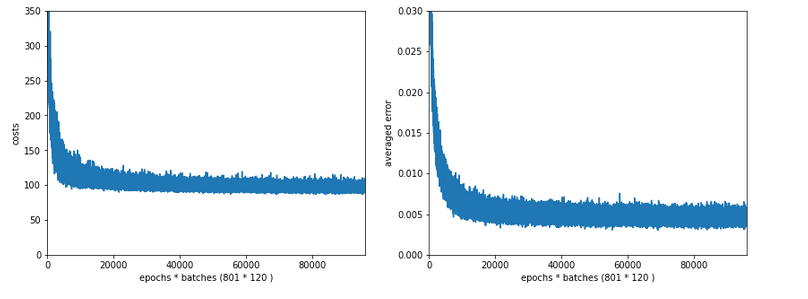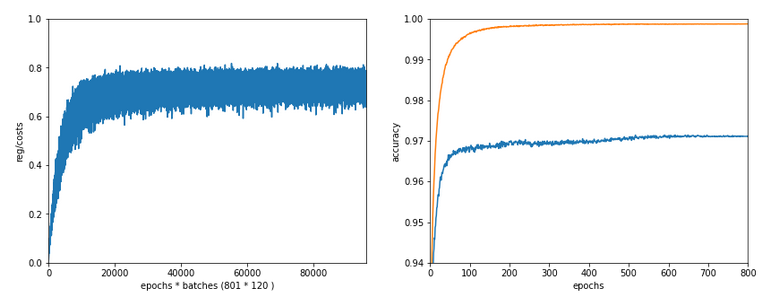We see that the regularization term actually dominates the total loss of a mini-batch at convergence. At least with our present parameter setting. In comparisoin to the total loss of the full training set the contribution is of course much smaller and typically below 1%.

# A small Lambda term

Let us reduce the regularization term via setting Lambda = 0.01. We expect its initial contribution to the costs of a batch to be smaller then, but this does NOT mean that the ratio to the standard costs of the batch automatically shrinks significantly, too:

Lambda2 = 0.01
Results: acc_train: 1.0 , acc_test: 0.9656, convergence after ca. 350 epochs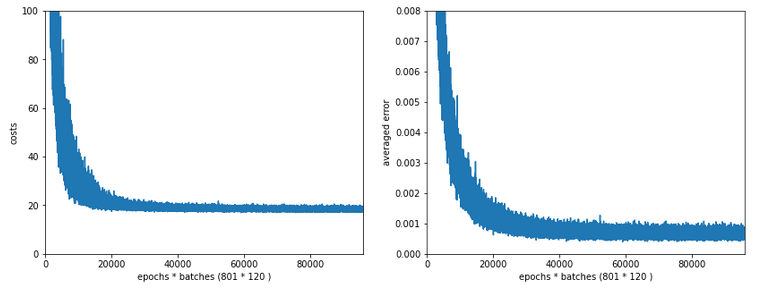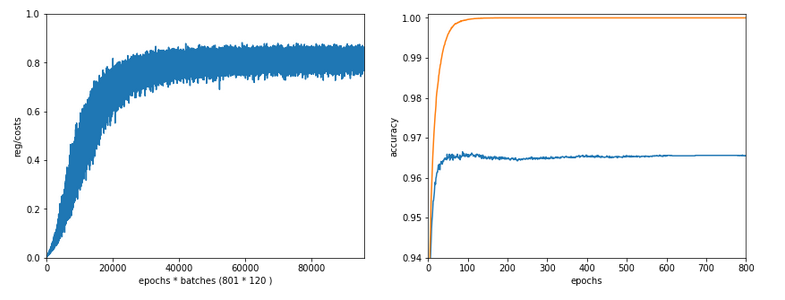Note the absolute scale of the costs in the plots! We ended up at a much lower level of the total loss of a batch! But the relative dominance of regularization at the point of convergence actually increased! However, this did not help us with the accuracy of our MLP-algorithm on the test data set - although we perfectly fit the training set by a 100% accuracy.

In the end this is what regularization is all about. We do not want a total overfitting, a perfect adaption of the grid to the training set. It will not help in the sense of getting a better general accuracy on other input data. A Lambda2 of 0.01 is much too small in our case!

# Slightly bigger regularization with Lambda2 = 0.2

So lets enlarge Lambda2 a bit:
Lambda2 = 0.2
Results: acc_train: 0.9946 , acc_test: 0.9728, convergence after ca. 700 epochs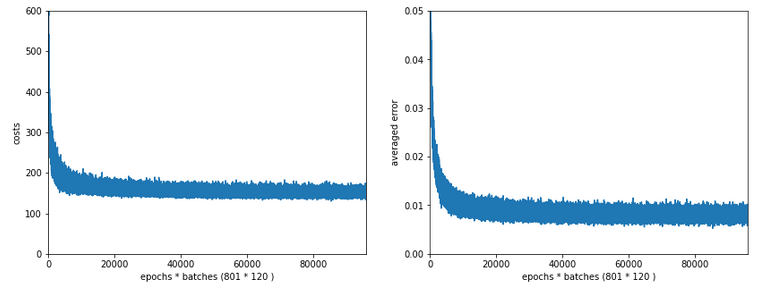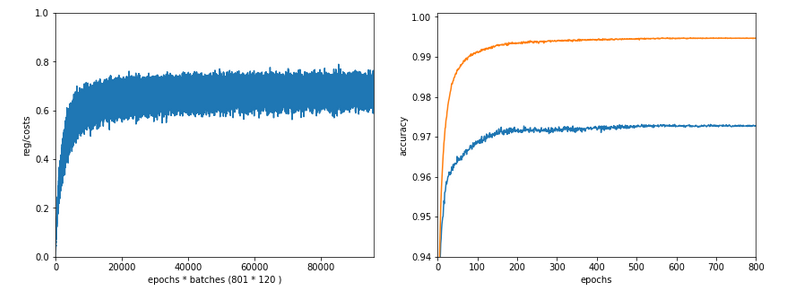We get an improved accuracy!

# Two other cases with significantly bigger Lambda2

Lambda2 = 0.4
Results: acc_train: 0.9858 , acc_test: 0.9693, convergence after ca. 600 epochs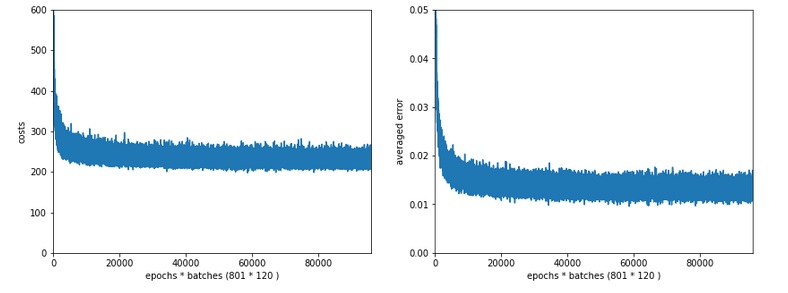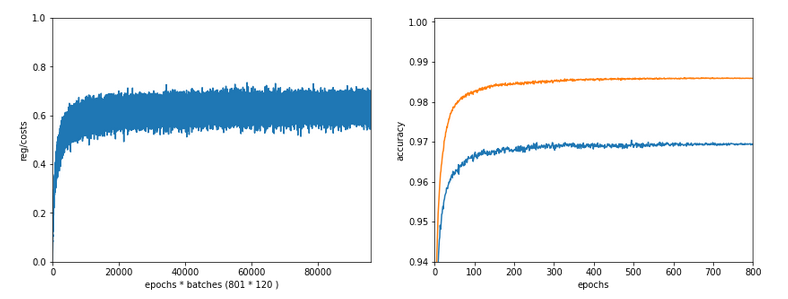Lambda2 = 0.8
Results: acc_train: 0.9705 , acc_test: 0.9588, convergence after ca. 400 epochs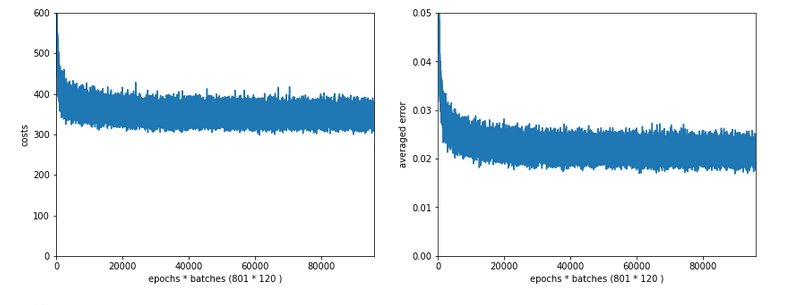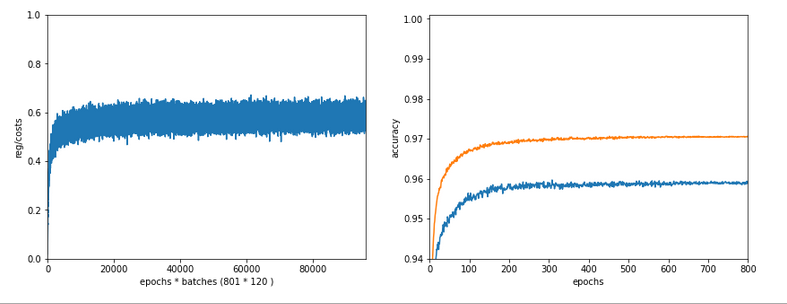OK, but in both cases we see a significant and systematic trend towards reduced accuracy values on the test data set with growing Lambda2-values > 0.2 for our chosen mini-batch size (500 samples).

# Conclusion

We learned a bit about the impact of regularization today. Whatever the exact Lambda2-value - in the end the contribution of a regularization term becomes a significant part of the total loss of a mini-batch when we approached the total cost minimum. However, the factor Lambda2 must be chosen with a reasonable size to get an impact of regularization on the final minimum position in the weight-space! But then it will help to improve accuracy on general input data in comparison to overfitted solutions!

But we also saw that there is some balance to take care of: For an optimum of generalization AND accuracy you should neither make Lambda2 too small nor too big. In our case Lambda2 = 0.2 seems to be a reasonable and good choice. Might be different with other datasets.

All in all studying the impact of a variation of achieved accuracy with the factor for a Ridge regularization term seems to be a good investment of time in ML projects. We shall come back to this point already in the next articles of this series.

In the next article

A simple Python program for an ANN to cover the MNIST dataset – XIV – cluster detection in feature space

we shall start to work on cluster detection in the feature space of the MNIST data before using gradient descent.